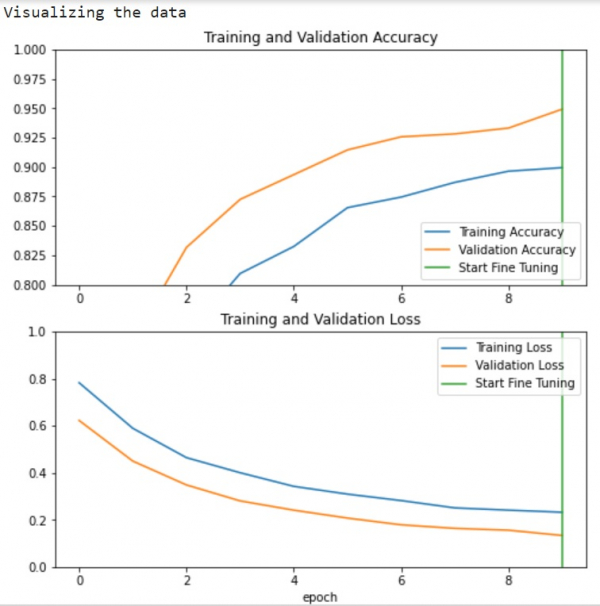# How can Tensorflow and pre-trained model be used to visualize the data using Python?

Tensorflow and the pre-trained model can be used to visualize the data using the ‘matplotlib’ library. The ‘plot’ method is used to plot the data on the console.

A neural network that contains at least one layer is known as a convolutional layer. We can use the Convolutional Neural Network to build learning model.

We will understand how to classify images of cats and dogs with the help of transfer learning from a pre-trained network. The intuition behind transfer learning for image classification is, if a model is trained on a large and general dataset, this model can be used to effectively serve as a generic model for the visual world. It would have learned the feature maps, which means the user won’t have to start from scratch by training a large model on a large dataset.

We are using the Google Colaboratory to run the below code. Google Colab or Colaboratory helps run Python code over the browser and requires zero configuration and free access to GPUs (Graphical Processing Units). Colaboratory has been built on top of Jupyter Notebook.

## Example

print("Visualizing the data")
plt.figure(figsize=(8, 8))
plt.subplot(2, 1, 1)
plt.plot(acc, label='Training Accuracy')
plt.plot(val_acc, label='Validation Accuracy')
plt.ylim([0.8, 1])
plt.plot([initial_epochs-1,initial_epochs-1], plt.ylim(), label='Start Fine Tuning')
plt.legend(loc='lower right')
plt.title('Training and Validation Accuracy')
plt.subplot(2, 1, 2)
plt.plot(loss, label='Training Loss')
plt.plot(val_loss, label='Validation Loss')
plt.ylim([0, 1.0])
plt.plot([initial_epochs-1,initial_epochs-1], plt.ylim(), label='Start Fine Tuning')
plt.legend(loc='upper right')
plt.title('Training and Validation Loss')
plt.xlabel('epoch')
plt.show()

## Output## Explanation

• The learning curves of the training and validation accuracy/loss is visualized.

• This is done once the fine-tuning is performed.

• The validation loss is higher than the training loss, which means there would be some overfitting.

• This overfitting could also be due to the fact that the training dataset is relatively smaller and similar to original MobileNet V2 datasets.

• Once fine tuning is done, model reaches 98% accuracy on the validation set.